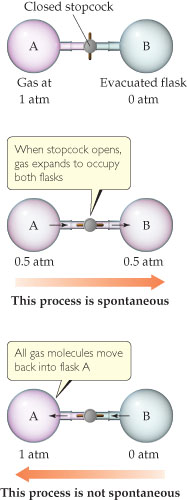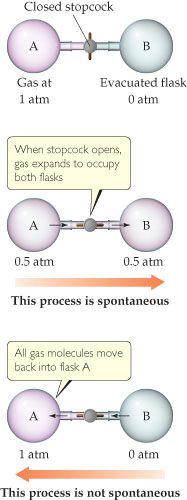# Problem: Expansion of a gas into an evacuated space is a spontaneous process. The reverse process-gas molecules initially distributed evenly in two flasks all moving into one flask-is not spontaneous.If flask B were smaller than flask A, would the final pressure after the stopcock is opened be greater than, equal to, or less than 0.5 atm?

###### FREE Expert Solution

We are given the following figure:We are asked if flask B were smaller than flask A, would the final pressure after the stopcock is opened be greater than, equal to, or less than 0.5 atm.

Recall that the ideal gas law is:

$\overline{){\mathbf{PV}}{\mathbf{=}}{\mathbf{nRT}}}$

The pressure and volume of a gas are related to the number of moles, gas constant and temperatureThe value nRT is constant

84% (263 ratings)###### Problem DetailsExpansion of a gas into an evacuated space is a spontaneous process. The reverse process-gas molecules initially distributed evenly in two flasks all moving into one flask-is not spontaneous.

If flask B were smaller than flask A, would the final pressure after the stopcock is opened be greater than, equal to, or less than 0.5 atm?